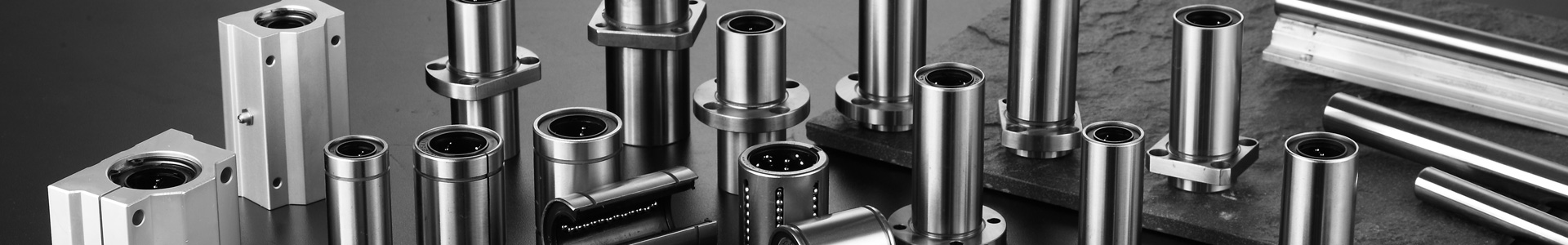• #### L

Product parameters
PDF inquiry Bearing Number Shaft diameter Basic load rating Weight(g) Dimensions(mm) Model Dimension mm Model Dimension mm Fit linear bearing Weight(g)
Rail units Rail units Slide units Slide units Dynamic
C N
Static
Co N
Slide units
（g）
Rails
(g)
D h2 H E θ W G A B T M S1xL J K S2 C N P h2 D W M G h1 θ K S1 I A Model Basic load rating
Dynamic
C N